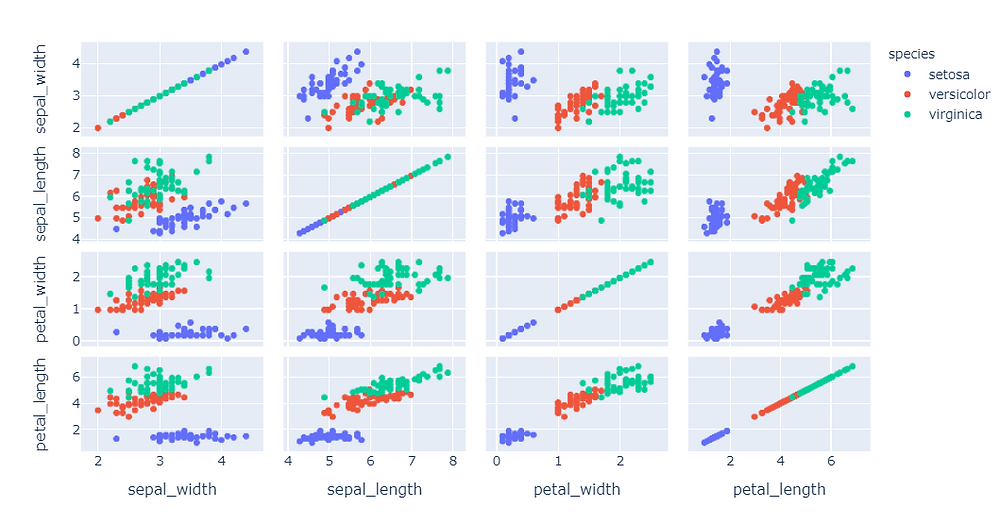Search

# Using TSNE to Visualize the Iris Dataset in 3D Using Python Machine Learning

Frist Load Iris dataset from sklearn and plot it into 2D:

2D Visualization

```#import plotly express
import plotly.express as px
df = px.data.iris()
#Select the features
features = ["sepal_width", "sepal_length", "petal_width", "petal_length"]
#plot scatter plot
fig = px.scatter_matrix(df, dimensions=features, color="species")
fig.show()```

Output:3D Visualization

```#import libraries
from sklearn.manifold import TSNE
import plotly.express as px
df = px.data.iris()
#Select the features
features = df.loc[:, :'petal_width']
#Fit into the model
tsne = TSNE(n_components=3, random_state=0)
projections = tsne.fit_transform(features, )
#Visualize
fig = px.scatter_3d(
projections, x=0, y=1, z=2,
color=df.species, labels={'color': 'species'}
)
fig.update_traces(marker_size=8)
fig.show()```

Output:At Realcode4you you can get all visualization related help which is related to any programming Languages like Python, R, MATLAB and more others.

realcode4you@gmail.com and get instant help with an affordable price.

### You Can Get Help In

• Python Visualization Help

• R Visualization Help

• Tableau Visualization Help

• D3.js Visualization Help

• Power BI Visualization Help

• And More Other Visualization Related help